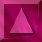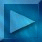Next : What is the problem? Notations

 Introduction

 A b s t r a c t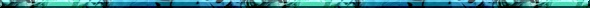Satellite images can be corrupted by an optical blur and electronic noise, due to the physics of the sensors. The degradation model is supposed to be known : blurring is modeled by convolution, with a linear operator H, and the noise is supposed to be additive, white and Gaussian, with a known variance. The recovery problem is ill-posed and therefore must be regularized. We use a regularization model which introduces a Phi function, which avoids noise amplification while preserving image discontinuities (ie. edges) of the restored image. This model exhibits two hyperparameters (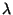and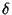). Our goal is to estimate the optimal parameters in order to automatically reconstruct images. Herein, we propose to use the Maximum Likelihood estimator, applied to the observed image. To optimize this criterion, we must estimate expectations by sampling (samples are extracted from a Markov chain) to evaluate its derivatives. These samples are images whose probability takes into account the convolution operator. Thus, it is very difficult to obtain them directly by using a standard sampler. We have developped a modified Geman-Yang algorithm, using an auxiliary variable and a cosine transform. We also present a new reconstruction method based on this sampling algorithm. Finally, we detail the MCMCML algorithm which enables to simultaneously estimateandparameters, and to reconstruct the corrupted image.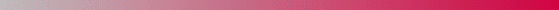André Jalobeanu - 24 / 8 / 1998